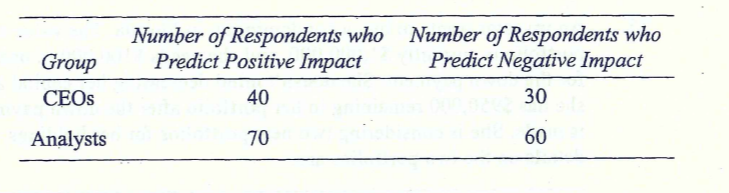Scan QR code or get instant email to install app

Question:

# What is the probability that a randomly selected individual from this group will be either an analyst or someone who thinks this legislation will have a positive impact on the economy? The following table summarizes the results of a poll taken of CEOs and analysts about the economic impact of a pending piece of legislation:A 0.85.
explanation

Using the addition rule for probabilities, P(analyst or positive) = P(analyst) + P(positive) - P(analyst and positive). P(A or positive) = 130 / 200 + 110/ 200 - (70 / 200) = 0.65 + 0. - 0.3\$ = 0.85. Alternatively, CEOs that predict positive impact = 40, analysts = 130, Prob (CEO positive or analyst) = (40 + 130) / 200 = 85%